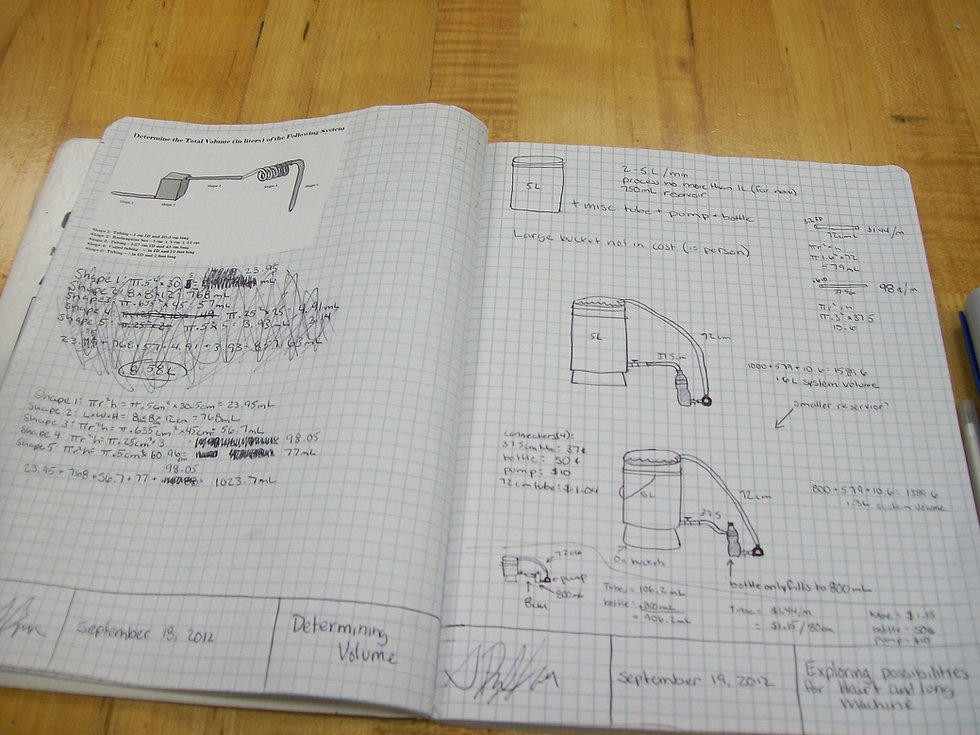Engineering Knowledge: Engineering Mathematics## Engineering Performance Matrix

Calculus is a branch of mathematics that focuses on understanding the changes between values that are related by functions of time. This involves determining how something changes, or how items add up, by breaking them into really tiny pieces. There are two different divisions of calculus; (1) differential calculus which focuses on calculating how things change from one moment to the next by dividing it in small fragments, and (2) integral calculus which focuses on understanding how much of something there is by piecing small fragments together. This area of mathematics is important to Engineering Literacy as engineering professionals frequently select and use calculus content and practices in the analysis and design of solutions to engineering problems. For example, the related mathematical applications can help one to accurately and efficiently calculate quantities like rates of flow of water from a tunnel or the rate of decay of a radioactive chemical.

## Performance Goal for High School Learners

I can, when appropriate, draw upon the knowledge of calculus content and practices such as (1) derivatives, (2) integrals, (3) differential and integral equations, and (4) vectors including dot and cross products, to solve problems in a manner that is analytical, predictive, repeatable, and practical.

## DERIVATIVES

I can compute derivatives of a given function formula through an identified calculus technique.

## INTEGRALS

I can compute integrals of a given function formula through an identified calculus technique.

I can select and apply the correct calculus technique to compute derivatives of a given function formula.

I can select and apply the correct calculus technique to compute integrals of a given function formula.

I can develop a function formula for the engineering problem at hand and then compute derivatives for the formula through the correct calculus technique.

I can develop a function formula for the engineering problem at hand and then compute integrals for the formula through the correct calculus technique.

## DIFFERENTIAL EQUATIONS

I can recognize when I need to apply differential and multivariable calculus equations.

I can correctly select and apply the correct differential equation and multivariable calculus technique for the engineering problem at hand.

I can extrapolate and justify the use of differential equations and multivariable calculus techniques to a variety of engineering problems.

## VECTORS (DOT PRODUCT & CROSS PRODUCT)

I can describe the basic algebraic operations in vector calculus (e.g. addition, subtraction, dot product, cross product).

I can explain properties of the dot and cross products, using mathematical descriptions.

I can develop a formula for the engineering problem at hand and then compute a vector field for the formula through the correct calculus technique.